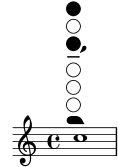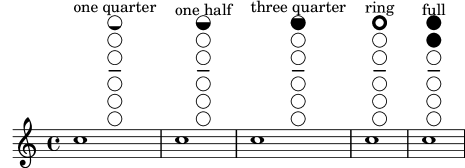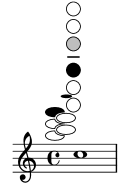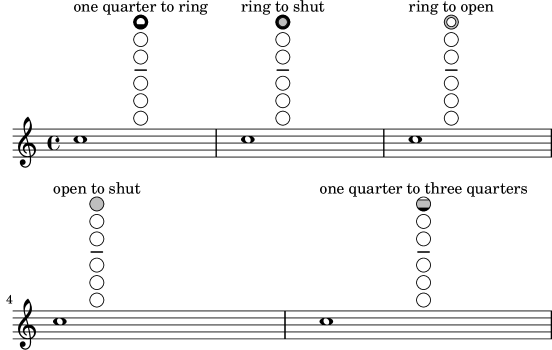#### 2.6.3.1 Woodwind diagrams

Woodwind diagrams can be used to indicate the fingering to be used for specific notes and are available for the following instruments:

• piccolo
• flute
• oboe
• clarinet
• bass clarinet
• saxophone
• bassoon
• contrabassoon

Woodwind diagrams are created as markups:

```c''1^\markup {
\woodwind-diagram #'piccolo #'((lh . (gis))
(cc . (one three))
(rh . (ees)))
}
```Keys can be open, partially-covered, ring-depressed, or fully covered:

```\textLengthOn
c''1^\markup {
\center-column {
"one quarter"
\woodwind-diagram #'flute #'((cc . (one1q))
(lh . ())
(rh . ()))
}
}

c''1^\markup {
\center-column {
"one half"
\woodwind-diagram #'flute #'((cc . (one1h))
(lh . ())
(rh . ()))
}
}

c''1^\markup {
\center-column {
"three quarter"
\woodwind-diagram #'flute #'((cc . (one3q))
(lh . ())
(rh . ()))
}
}

c''1^\markup {
\center-column {
"ring"
\woodwind-diagram #'flute #'((cc . (oneR))
(lh . ())
(rh . ()))
}
}

c''1^\markup {
\center-column {
"full"
\woodwind-diagram #'flute #'((cc . (oneF two))
(lh . ())
(rh . ()))
}
}
```Trills are indicated as shaded keys:

```c''1^\markup {
\woodwind-diagram #'bass-clarinet
#'((cc . (threeT four))
(lh . ())
(rh . (b fis)))
}
```A variety of trills can be displayed:

```\textLengthOn
c''1^\markup {
\center-column {
"one quarter to ring"
\woodwind-diagram #'flute #'((cc . (one1qTR))
(lh . ())
(rh . ()))
}
}

c''1^\markup {
\center-column {
"ring to shut"
\woodwind-diagram #'flute #'((cc . (oneTR))
(lh . ())
(rh . ()))
}
}

c''1^\markup {
\center-column {
"ring to open"
\woodwind-diagram #'flute #'((cc . (oneRT))
(lh . ())
(rh . ()))
}
}

c''1^\markup {
\center-column {
"open to shut"
\woodwind-diagram #'flute #'((cc . (oneT))
(lh . ())
(rh . ()))
}
}

c''1^\markup {
\center-column {
"one quarter to three quarters"
\woodwind-diagram #'flute #'((cc . (one1qT3q))
(lh . ())
(rh . ()))
}
}
```The list of all possible keys and settings for a given instrument can be displayed on the console using `#(print-keys-verbose 'flute)` or in the log file using `#(print-keys-verbose 'flute (current-error-port))`, although they will not show up in the music output.

Creating new diagrams is possible, although this will require Scheme ability and may not be accessible to all users. The patterns for the diagrams are in files ‘scm/define-woodwind-diagrams.scm’ and ‘scm/display-woodwind-diagrams.scm’.

#### Woodwind diagrams listing

The following music shows all of the woodwind diagrams currently defined in LilyPond.

```\layout {
indent = 0
}

\relative c' {
\textLengthOn
c1^
\markup {
\center-column {
'tin-whistle
" "
\woodwind-diagram
#'tin-whistle
#'()
}
}

c1^
\markup {
\center-column {
'piccolo
" "
\woodwind-diagram
#'piccolo
#'()
}
}

c1^
\markup {
\center-column {
'flute
" "
\woodwind-diagram
#'flute
#'()
}
}
c1^\markup {
\center-column {
'oboe
" "
\woodwind-diagram
#'oboe
#'()
}
}

c1^\markup {
\center-column {
'clarinet
" "
\woodwind-diagram
#'clarinet
#'()
}
}

c1^\markup {
\center-column {
'bass-clarinet
" "
\woodwind-diagram
#'bass-clarinet
#'()
}
}

c1^\markup {
\center-column {
'saxophone
" "
\woodwind-diagram
#'saxophone
#'()
}
}

c1^\markup {
\center-column {
'bassoon
" "
\woodwind-diagram
#'bassoon
#'()
}
}

c1^\markup {
\center-column {
'contrabassoon
" "
\woodwind-diagram
#'contrabassoon
#'()
}
}
}
```#### Graphical and text woodwind diagrams

In many cases, the keys other than the central column can be displayed by key name as well as by graphical means.

```\relative c'' {
\textLengthOn
c1^\markup
\woodwind-diagram
#'piccolo
#'((cc . (one three))
(lh . (gis))
(rh . (ees)))

c^\markup
\override #'(graphical . #f) {
\woodwind-diagram
#'piccolo
#'((cc . (one three))
(lh . (gis))
(rh . (ees)))
}
}
```#### Changing the size of woodwind diagrams

The size and thickness of woodwind diagrams can be changed.

```\relative c'' {
\textLengthOn
c1^\markup
\woodwind-diagram
#'piccolo
#'()

c^\markup
\override #'(size . 1.5) {
\woodwind-diagram
#'piccolo
#'()
}
c^\markup
\override #'(thickness . 0.15) {
\woodwind-diagram
#'piccolo
#'()
}
}
```#### Woodwind diagrams key lists

The snippet below produces a list of all possible keys and key settings for woodwind diagrams as defined in `scm/define-woodwind-diagrams.scm`. The list will be displayed in the log file, but not in the music. If output to the console is wanted, omit the `(current-error-port)` from the commands.

```#(print-keys-verbose 'piccolo (current-error-port))
#(print-keys-verbose 'flute (current-error-port))
#(print-keys-verbose 'flute-b-extension (current-error-port))
#(print-keys-verbose 'tin-whistle (current-error-port))
#(print-keys-verbose 'oboe (current-error-port))
#(print-keys-verbose 'clarinet (current-error-port))
#(print-keys-verbose 'bass-clarinet (current-error-port))
#(print-keys-verbose 'low-bass-clarinet (current-error-port))
#(print-keys-verbose 'saxophone (current-error-port))
#(print-keys-verbose 'soprano-saxophone (current-error-port))
#(print-keys-verbose 'alto-saxophone (current-error-port))
#(print-keys-verbose 'tenor-saxophone (current-error-port))
#(print-keys-verbose 'baritone-saxophone (current-error-port))
#(print-keys-verbose 'bassoon (current-error-port))
#(print-keys-verbose 'contrabassoon (current-error-port))

\score {c''1}
```